﻿ Peter Shor's algorithm in quantum computer is impractical

# Quantum computer is a scam.

Top    IBM is not quantum !

## Parallel worlds in quantum computer.

### [ Nature, MIT are spreading unreal quantum computer. ]

(Fig.1)  How "fantasy" quantum computer spread ?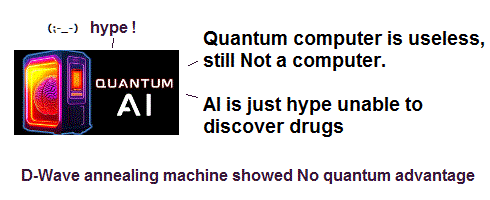David Deutsch first proposed quantum computer which could calculate many things at the same time using parallel worlds.

Because the present quantum mechanics is based on "superposition" where a single particle can be everywhere at the same time, until we observe it.

Of course, it's impossible to see these parallel worlds, so this quantum computer is impracticable, forever.

But under useless physics, they crave some imaginary target to collect money.
So, commercial top journal Nature (+ bbc ) started spreading this fantasy.

In US, commercial MIT college takes the lead in quantum computer and parallel worlds to collect money and justify their tuition.

Now, many corporations such as Google are deceived into wasting money in "pie-in-the-sky" quantum computer.

## Classical (= ordinary ) computer.

### [ Classical computer calculates "once" at the same time. ]

(Fig.2)  Ordinary computer calculates in order.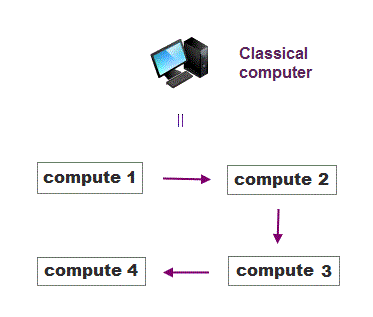In a conventional classical computer, we can compute one thing at the same time with a single machine.

When there are four things to calculate, classical computer does computing in order from 1 to 4.

## Parallel computing by quantum computer ?

### [ Quantum computer gets all possible results at the same time ? ]

(Fig.3)  ↓ This fantasy quantum computer is really practicable ?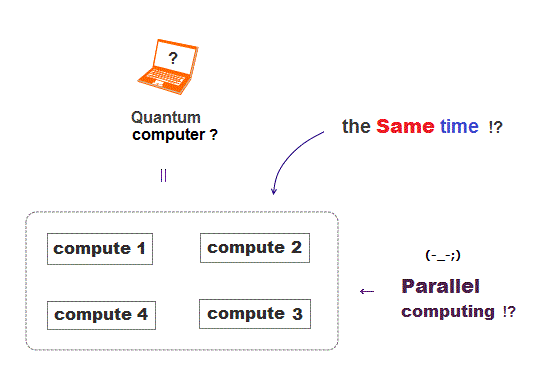They say quantum computer can compute all possible results at the same time using parallel different worlds.  See this.

Quantum mechanics claims each computer bit can be in two different states ( 0 and 1 ) at the same time, which is called superposition.

So they claim one single operation is enough to perform all different possible computations instantly in quantum computer.

Unfortunately, this idea is a too optimistic delusion.  This is the reason quantum computer is impracticable despite longtime researches..

## Parallel computing in many worlds.

### [ Computing all possible values at the same time ? ]

(Fig.4)  Quantum computer depends on parallel worlds.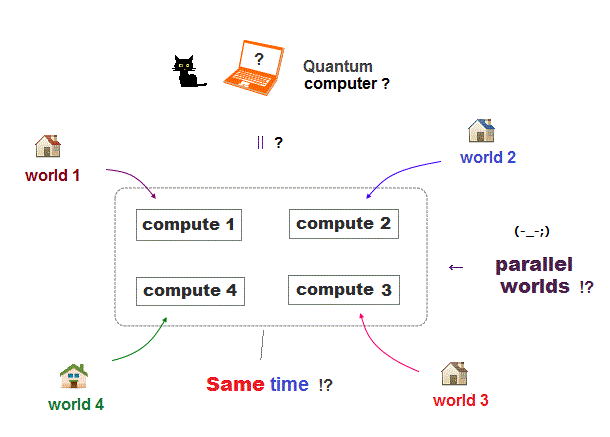The basic idea of quantum computer is to utilize parallel worlds to compute different values at the same time.

So if we can realize ultrafast quantum computer using parallel computing someday, it will mean the proof of unrealistic parallel worlds.

Unfortunately, the concept of parallel world is just fiction, so quantum computer is impracticable, forever.

## Dead and alive at the same time ?

### [ Quantum cat can be dead and alive at the same time ? ]

(Fig.5)  Quantum superposition = Schrödinger's cat ?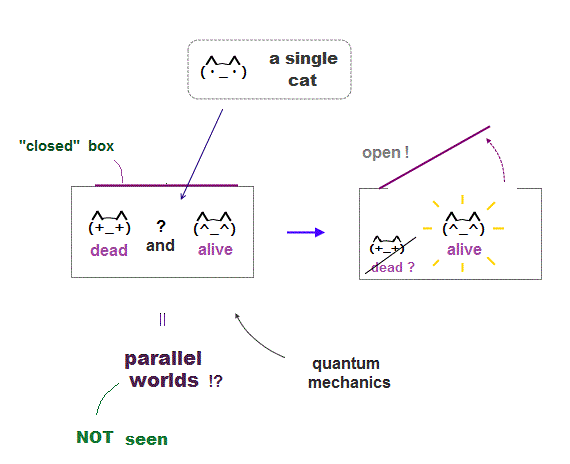Quantum mechanics relies on fantasy "superposition" where each object can be in two different states at the same time ?

It's called "Schrodinger's cat, where a single cat is both dead and alive until someone opnes the box to find out.

As you feel, it's completely impossible for each cat to be both dead and alive at the same time.  So quantum mechanics lacks reality.

The point is we cannot see parallel different worlds directly, because it collapses into a single world, the moment we observe it.

So superposition = parallel worlds, the heart of quantum mechanics has No evidence, it's too good to be true.

## Mistake "alternating" for parallel worlds.

### [ An atom just alternates between ground and excidted states ! ]

(Fig.6)  But quantum mechanics mistakes it for "parallel worlds" !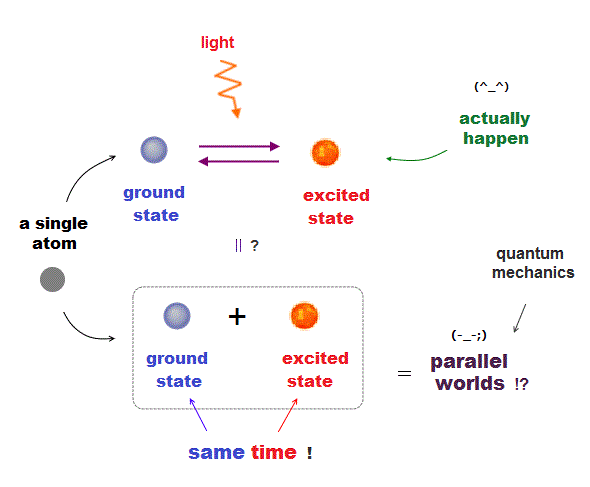Though parallel worlds clearly lack reality, why do we see its news and paper very often ?

The point is the present physics misinterpret some "classical phenomena" as "parallel worlds".  Of course, this is an "intentional" mistake.

In Fig.6, a single atom is alternating between ground state and excited state by laser light.  This is a realistic (= classical ) phenomenon.

But they intentionally mistake "this alternating" for "superposition" where two different ground and excited states coexit at the same time.

And when we try to observe the atom, it "collapses" to one of ground or excited states.  So we cannot directly confirm parallel worlds.

It's too good to be true.  See this Fig.6  and this.

## D-Wave is NOT a quantum computer.

### [ D-Wave uses only "classical" phenomena. ]

(Fig.7)  D-Wave proved to be NOT-faster.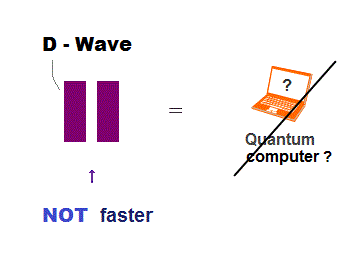D-Wave is a hot topic in the present quantum computer.
Recently, D-Wave machine proved to be NOT faster than classic PC.

The important point is that D-Wave is NOT a quantum computer, but a classical machine.  So it can neither be faster nor useful.

Of course, if they changed the definition of "quantum", it may be called (fake) "quantum computer".  But doubtful.

## How does D-Wave computer work ?

### [ D-Wave is just finding "the lowest energy state". ]

(Fig.8)  Moving electrons gradually settle in ground state.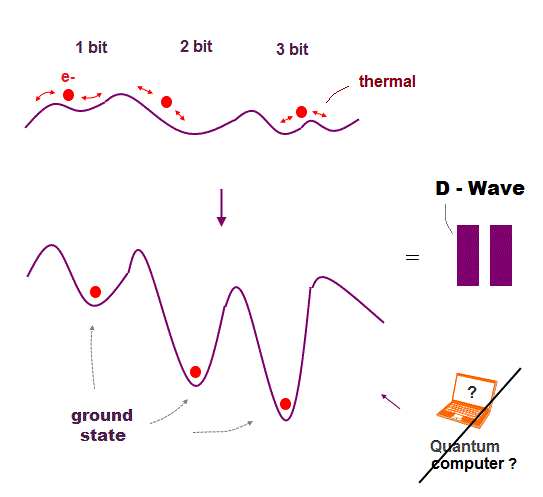It is often said that D-Wave machine is NOT a real quantum computer.
Because D-Wave machine does NOT use parallel computing.

D-Wave just finds the lowest-energy ground state depending on initial setting of parameters through annealing.

Though D-Wave claims this is due to quantum tunnel, its effect is too small to confirm.

We can say it is due to other thermal noises or nonspecific oscillation.

## D-Wave is just a "classical" computer.

### [ Because it doesn't use parallel computing. ]

(Fig.9)  The difference between D-Wave and quantum computer.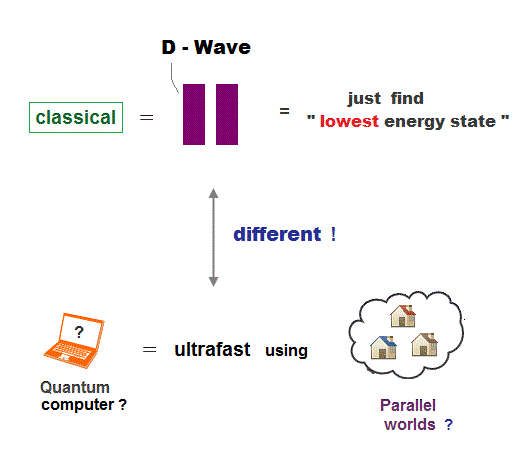In D-Wave machine, each electron gradually settles in its most stable (= lowest-energy ) state, which pattern can be freely adjustable.

So this D-Wave machine is completely different from "real" quantum computer, which perform parallel computing in parallel worlds.

D-Wave does NOT perform any computing.  It just finds the most stable ground state.  "Simultaneous computation" does not happen in D-Wave.

So it may be used only in some special optimization such as "traveling salesman problem" ( in classical way ).

D-Wave has nothing to do with "parallel-world computing", so it can never be ultra-fast quantum computer.

## Factorization in prime numbers.

### [ Quantum computer outperforms classical computer ? ]

(Fig.10)  Only factorization 15 = 3 × 5 can be done ?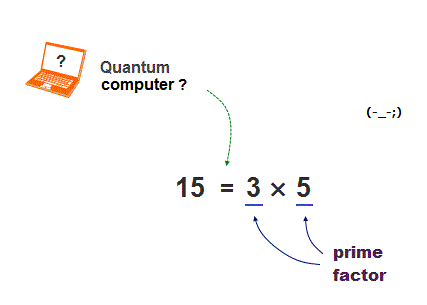It is said that quantum computer can find prime factors much faster than classical computer.  But it is a big lie.

They could only factor 15 into 3 × 5, so far.
Furthermore, it causes 50 % error !  So completely useless.

There is NO proof that a quantum computer factored some numbers faster than classical computer.

## Shor's algorithm for quantum factorization.

### [ Classical computer compute one value at the same time. ]

(Fig.11)  When classical computer calculates four times,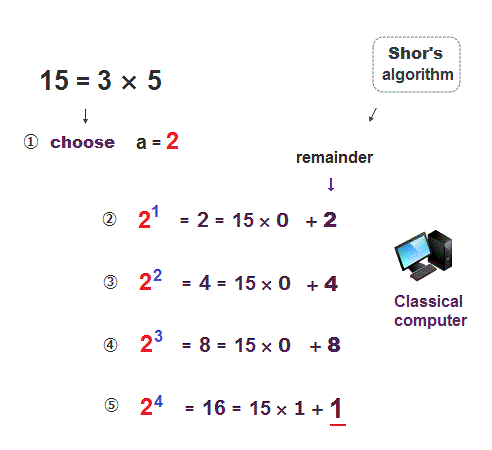Factorization into prime numbers can be performed using Shor's algorithm.  Here we factor 15 into 3 × 5 using Shor's algorithm.

First, we choose an arbitrary number ( here, we choose "2" ).
Seond, we devide 2 (= 21 ) by 15, and its remainder is 2.

In the same way, after we get 2 squared (= 22 ), 2 cubed (= 23 ) and 2 to the power of four (= 24 ), we calculate each remainder by dividing them by 15.

Continue until the remainder becomes "1".

## Shor's algorithm ---- continued.

(Fig.12)   Finally, we get 15 = 3 × 5.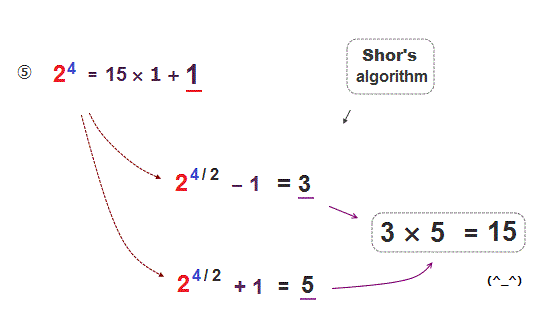When 24 (= 16 ) is divided by 15, its remainder becomes 1.
Then we calculate 24/2 (= 2 squared ) ± 1

These values are equal to 3 and 5.
So we can factor 15 into 3 × 5.

## Quantum computer calculates at the same time !?

### [ Parallel computing four different values at the same time !? ]

(Fig.13)  It can get four different remainders at the same time ?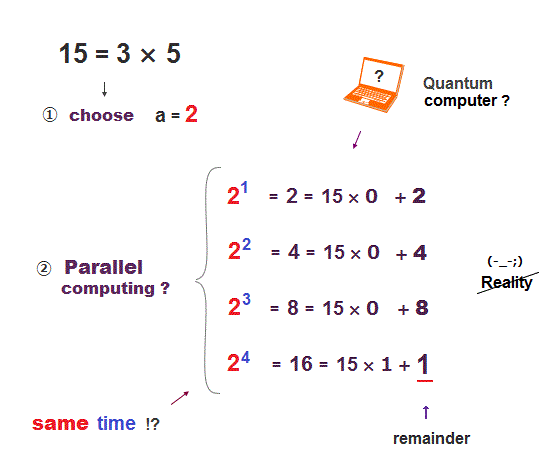Classical computer has to calculate four times to get four different remainders.

Surprisingly, they argue that quantum computer can calculate these four different remainders at the same time using parallel worlds !

This is the reason why physicists claim quantum computer can calculate many different values much faster than classical computer.

But of course, this computing using paralell worlds is just "fantasy".

## Classical computer  4 bits.

### [ Different patterns at different times classical PC. ]

(Fig.14)  Each bit is white (= 0 ) or black (= 1 )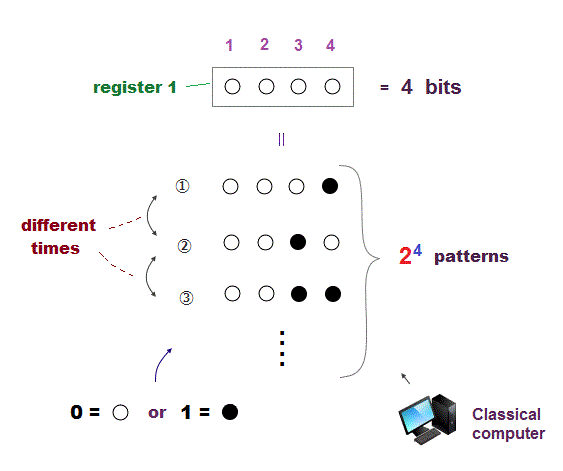There are four bits in classical computer in Fig.14.
Each bit can be white (= 0 ) or black (= 1 ).

So there are 24 different patterns in four bits.
Different patterns cannot happen at the same time in classical PC.

## All different patterns happen at the same time ?

### [ Quantum computer bits are "superposition" of all different patterns ? ]

(Fig.15)  Quantum computer 4 bits = 24 parallel worlds !?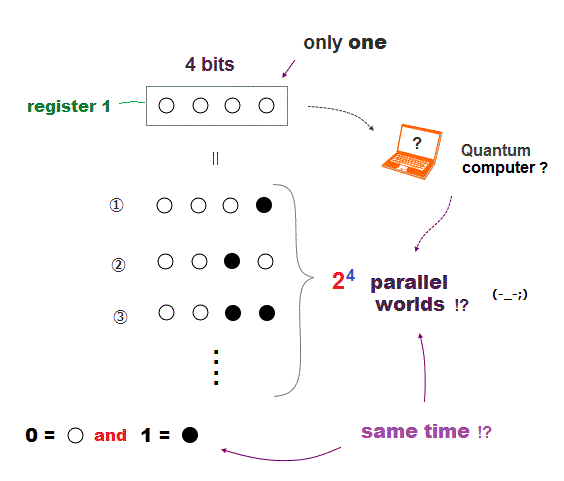How about unrealistic quantum computer ?
Quantum computer bits can be all possible states at the same time.

So when there are four quantum bits, all 24 = 16 different patterns are allowed to coexist at the same time in quantum computer !

It means there are 24 parallel worlds in these quantum bits at the same time.

## Quantum computer = Parallel computing ?

### [ Quantum computing different values at the same time !? ]

(Fig.16)  Different values coexist in each register ?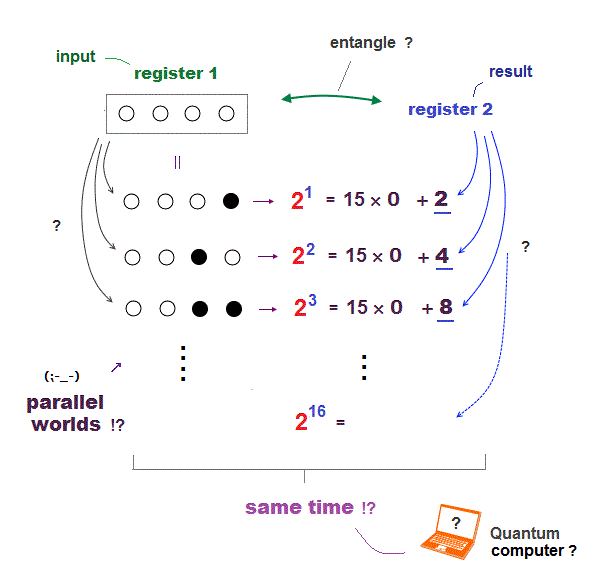There is a pair of register 1 and 2.  Different from classical computer, quantum computer calculates different values at the same time.

When executing Shor's algorism, a single register 1 contains 16 different patterns, and a register 2 contains each different results (= remainder ).

They claim all these different patterns happen at the same time in quantum computer !

## Parallel worlds in quantum registers.

### [ Each parallel world is entangled with another world ? ]

(Fig.17)  ↓ Parallel worlds in quantum computer registers.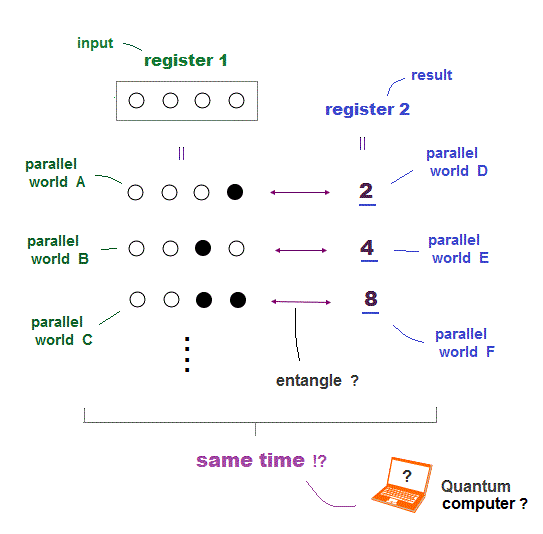They argue there are parallel different worlds in each register 1 and 2.
21 divided by 15 gives the remainder "2".

So parallel world A (= 21 ) in register 1 is entangled with parallel world D ( = 2 ) in register 2.

Of course, we cannot observe different parallel worlds, so these ideas are just imaginary delusions.

## Shor's algorithm in quantum computer

### [ Observing register 2 collapses register 1 into 4, 8, 12 .. ]

(Fig.18)  When remainder becomes "1", its period is "4"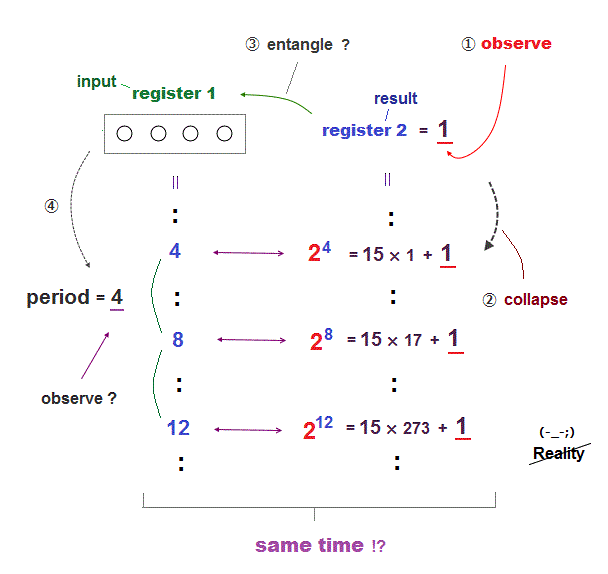In Shor's algorithm, we pick up the value, when the remainder is 1.
In quantum computer, we observe the case when the register 2 becomes "1".

In this case (= remainder is 1 ), the values of register 1 is either 4, 8 or 12 ..  After reading register 1 repeatedly, we know its period is "4".

See the method ( p.1 ).

(Fig.19)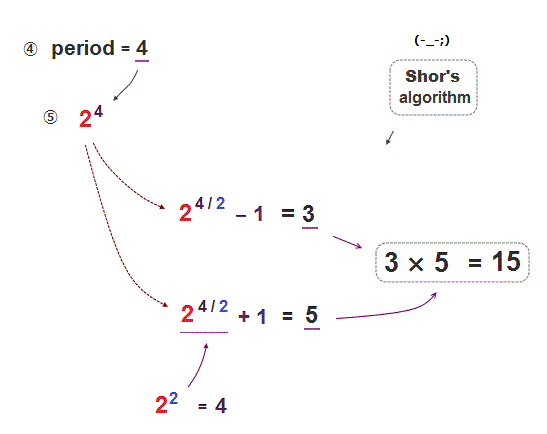Using the value "4" of the period in register 1, we can guess the prime factors 3 and 5.

If parallel worlds are real, this factorization can be perfomed much faster than classical computer.

But unfortunately, there are NO evidence for parallel worlds, so far.

## Quantum computer is impossible

### [ Science paper did NOT use parallel computing. ]

(Fig.20)  Quantum computer in Science didn't use Shor's algorithm.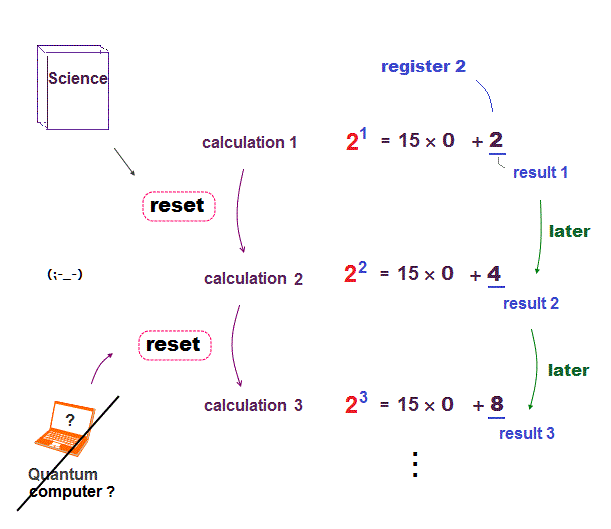A recent Science paper claims they used Shor's algorithm in quantum computer.

But in fact, this Science paper has nothing to do with quantum computer ( Shor's algorithm ).  See the critic who disproved this Science paper.

In the original paper (p.2), they adopted "semiclassical method" (← Not quantum ! ) and recycled each bit instead of parallel computing.

## Science paper is NOT quantum computing.

### [ They compute different values in order at different times. ]

(Fig.21)  Reset and recycle a single bit instead of parallel computing.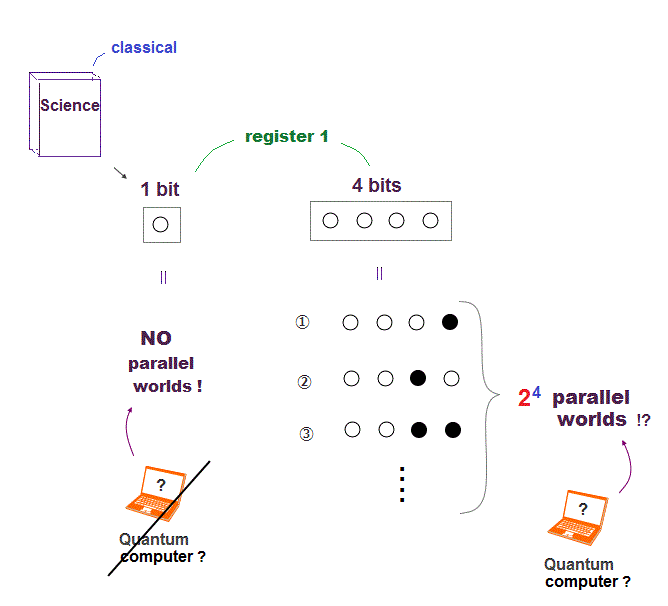In this Science paper, register 1 is only a single bit, which cannot contain parallel different worlds.

So each time they compute different remainder, they reset and recycle each bit before computing the next remainder.

This is the reason they call it "semiclassical" method.  So even in the simplest factorization 15 = 3 × 5, quantum computer is impossible.

It means they couldn't generate parallel worlds for parallel computing even in factoring 15 = 3 × 5.

So the world "scalable" by MIT physicists is completely misleading.
This is similar to the previous Nature photonics.

## Classical oscillation = Parallel worlds ?

### [ They mistake "classical oscillation" for superposition. ]

(Fig.22)  Alternate between upper and lower states = Parallel worlds ?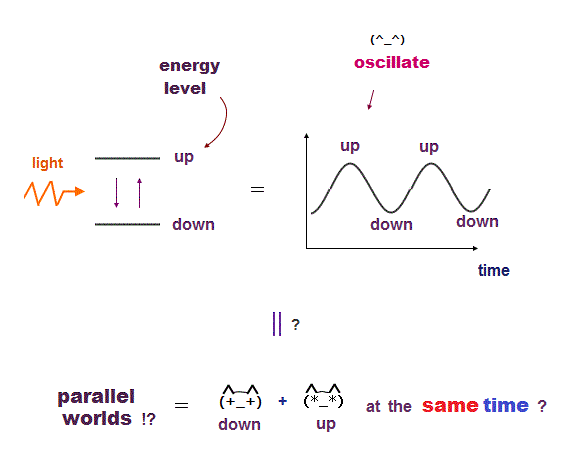We cannot observe a grotesque cat which can be dead and alive at the same time.  So parallel worlds are just fiction.

In spite of this, physicists try to create parallel worlds = superposition of two different states by force !

Suppose an atom is alternating between upper and lower energy states by laser light, which means just "classical oscillation".

But they intentionally mistake this "classical oscillation" for "parallel worlds" where the upper and lower states coexist at the same time !

This logic is ridiculous.

## Far-fethed quantum interpretation

### [ They misinterpret "classical" as "quantum worlds" ! ]

(Fig.23)  Alternating between two states = Two Parallel worlds !?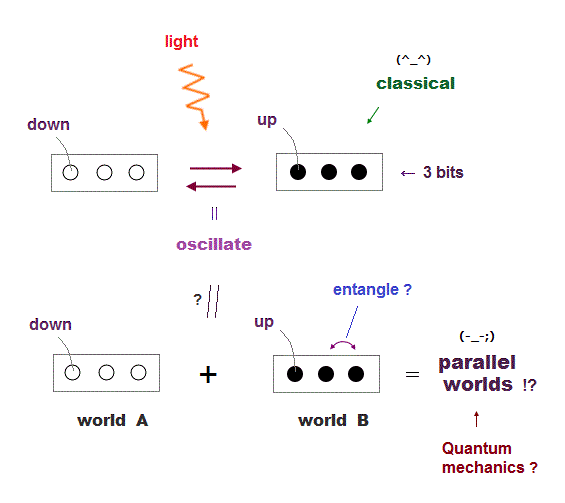Here we explain this Nature physics paper, which claims simple Shor's algorithm is performed.

They often use the concepts such as quantum superposition (= parallel worlds ) and entanglement.

Three computer bits are alternating between two different states by laser light in Fig.23.  This is just "classical oscillation".

But they intentionally misinterpret this classical oscillating as "parallel worlds", where each bit is entangled like white-white or black-black.

See this paper Fig.2.

## Shor's algorithm -- simple case

### [ Factoring 15 into 3 × 5 by Shor's algorithm. ]

(Fig.24)  Choosing "4" as an arbitrary number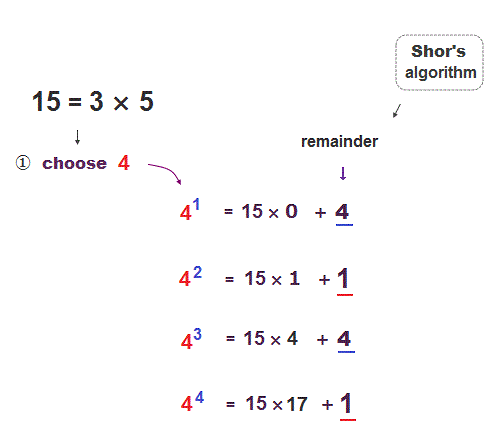Again we factor 15 into 3 × 5 using Shor's algorithm.
Here we pick up "4" as an arbitrary number.

When the remainder becomes "1", its period is "2".

(Fig.25)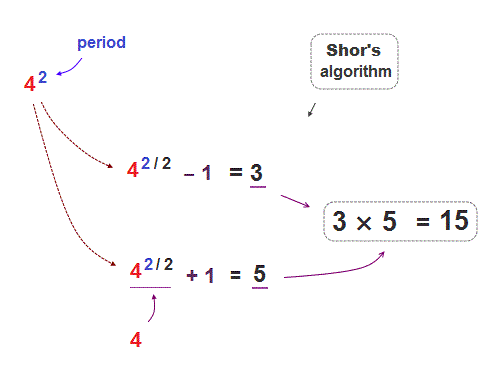As a result, we can get prime factors 3 and 5 by starting from "4".

## Shor's algorithm is performed ?

### [ Simplest Shor's case is calculated with three bits ? ]

(Fig.26)  ↓ Only two different patterns are needed. = classical.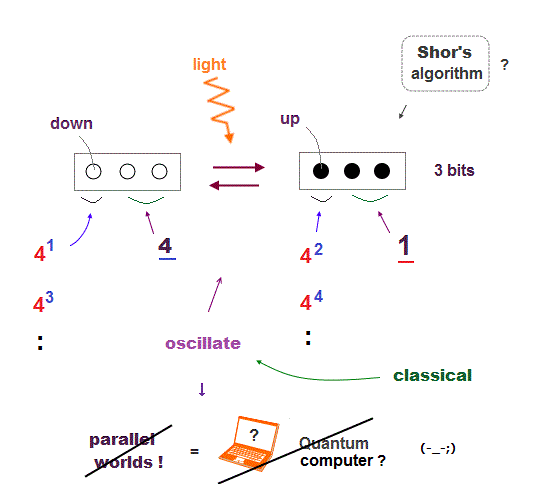When the period is 2, it's the simplest case.
We need to prepare only two different states in registers.

Of course, it means they did NOT compute factorization, because they just use the "already-known" answer starting from "4".

So this paper has nothing to do with quantum computer (= Shor's algorithm, this p.3 ).  They just use "classical oscillation" of bits' state.

In Fig.26, when the resister is "three whites", the remainder is 4, and when three blacks", the remainder is "1".  They just artificially defined it.

After all, these experiments proved that creating quantum superposition for parallel computing is impossible even in the simplest factorization !

## Conclusion:  Quantum computer is "scam".

### [ Parallel worlds and entanglement lack reality. ]

(Fig.27)  Schrodinger's cat cannot be dead and alive at the same time !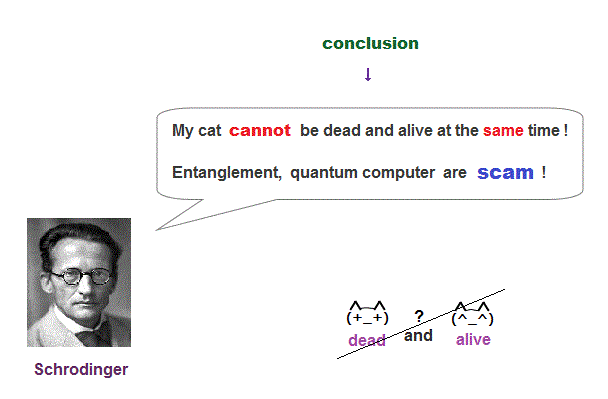The conclusion is parallel worlds are fiction, Schrodinger cat cannot be dead and alive at the same time.

It means quantum computer based on parallel computing is just a scam to defraud people and corporations of their money.

This miserable situation is what the present physics is.
All students in the world are deceived into destroying their careers.2016/3/11 updated. Feel free to link to this site.